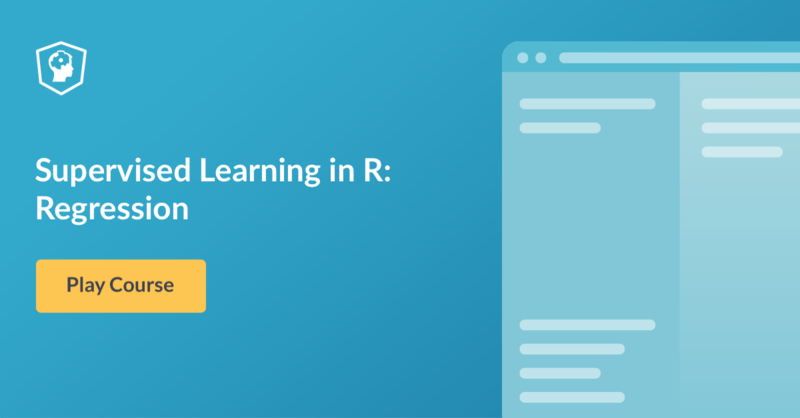# New Course - Supervised Learning in R: Regression

New course launched today! Learn how to train, evaluate and make predictions with different regression models. Continue your journey into machine learning.

Hello R users, new course hot off the press today by Nina Zumel - Supervised Learning in R: Regression!

From a machine learning perspective, regression is the task of predicting numerical outcomes from various inputs. In this course, you'll learn about different regression models, how to train these models in R, how to evaluate the models you train and use them to make predictions.

Take me to chapter 1!

Supervised Learning in R: Regression features interactive exercises that combine high-quality video, in-browser coding, and gamification for an engaging learning experience that will make you a master in supervised learning with R!What you'll learn:

Chapter 1: What is Regression?

In this chapter you are introduced to the concept of regression from a machine learning point of view. We present the fundamental regression method: linear regression. You will learn how to fit a linear regression model and to make predictions from that model.

Chapter 2: Training and Evaluating Regression Models

You will now learn how to evaluate how well your models perform. You will review how to evaluate a model graphically, and look at two basic metrics for regression models. You will also learn how to train a model that will perform well in the wild, not just on training data. Although we will demonstrate these techniques using linear regression, all these concepts apply to models fit with any regression algorithm.

Chapter 3: Issues to Consider

Before moving on to more sophisticated regression techniques, you will look at some other modeling issues: modeling with categorical inputs, interactions between variables, and when you might consider transforming inputs and outputs before modeling. While more sophisticated regression techniques manage some of these issues automatically, it's important to be aware of them, in order to understand which methods best handle various issues -- and which issues you must still manage yourself.

Chapter 4: Dealing with Non-Linear Responses

Now that you have mastered linear models, you will begin to look at techniques for modeling situations that don't meet the assumptions of linearity. This includes predicting probabilities and frequencies (values bounded between 0 and 1); predicting counts (nonnegative integer values, and associated rates); and responses that have a nonlinear but additive relationship to the inputs. These algorithms are variations on the standard linear model.

Chapter 5: Tree-Based Methods

In this chapter, you will look at modeling algorithms that do not assume linearity or additivity, and that can learn limited types of interactions among input variables. These algorithms are *tree-based* methods that work by combining ensembles of *decision trees* that are learned from the training data.

Get started with Supervised Learning in R: Regression today!